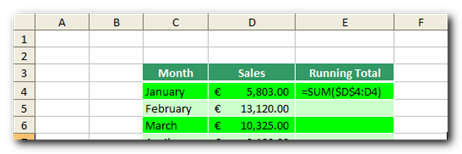If, as I expect, you have a list of figures and need to calculate a running total (aka cumulative sum), use this super quick Excel formula tip which uses the Excel SUM function and combines it with absolute cell references (e.g. \$B\$1).

The trick? Use the SUM function throughout the running total column by fixing the starting reference of your range, like so:Use AutoFill to copy this formula all the way down. With just one formula using the SUM function and a little AutoFill click-and-drag mouse action, you now have completed a running total (or cumulative sum, if you like big words).